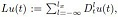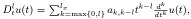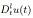##### A Simulation Study for the AIC and Likelihood Cross-validation: The Case of Exponential Versus Weibull Distributions

Kunio Takezawa

Journal of Advances in Mathematics and Computer Science, Page 1-13
DOI: 10.9734/JAMCS/2018/43344

Various methods are available for choosing statistical models. It is difficult to know which model selection criterion is the best for specific data. This paper discusses a method for choosing the model selection criterion based on the characteristics of the data and models. As an example, we examined the choice between AIC and likelihood cross-validation as the model selection criterion with the exponential distribution and Weibull distribution as candidate models. First, we examined the characteristics of AIC and likelihood cross-validation using data generated from an exponential distribution or Weibull distribution; AIC and likelihood cross-validation show substantially different natures. Next, from the results of the numerical simulations, we propose an intuitive method for deciding whether to use AIC or likelihood cross-validation.

##### Multi-derivative Linear Multi-step Methods for Solving Third Order Boundary Value Problems

E. A. Areo, T. V. Abejide

Journal of Advances in Mathematics and Computer Science, Page 1-10
DOI: 10.9734/JAMCS/2018/42045

Multi-derivative linear multi-step methods with continuous coefficients was derived through the block method approach using power series as basis function. Discrete scheme systems involving the multi-derivative linear methods were developed and their basic properties examined. The resulting schemes were used to solve general third order boundary value problems in ordinary differential equations without reducing it to first order. Numerical results were compared with the existing methods to show the accuracy and efficiency of the method. Results obtained show that our methods performed better than the existing methods.

##### Acceleration in a Fundamental Bound State Theory and the Fate of Gravitational Systems

Hans-Peter Morsch

Journal of Advances in Mathematics and Computer Science, Page 1-13
DOI: 10.9734/JAMCS/2018/42590

The dynamics of a bound state theory - based on a QED like Lagrangian with fermions coupled to boson fields - has been studied explicitly. Different from the Hamilton approach studied earlier, an additional acceleration term is found, which is spurious for fundamental bound states. However, for composite systems of many particles this term drives individual particles to a coherent rotation, which lowers the kinetic energy and leads to a collapse.

Applied to gravitation - described by magnetic binding of lepton-hadron pairs - a self-consistent fit of the primary (e-p)2 bound state is obtained. Of importance, the acceleration term is quite large and drives composite systems to a collapse and complete annihilation. However, stable galactic objects are obtained, if the lowering of the kinetic energy is compensated by a reduction of binding. Of special interest, a ”matter-antimatter symmetric” system, composed of equal amounts of (ep+) and (e+p) pairs (or hydrogen and antihydrogen atoms), leads to a delayed and incomplete collapse, in which the matter-antimatter symmetry is broken due to the chiral structure of leptons.

##### Analysis of an Epidemic Model of Multiple Disease Transmission with Vaccination and Therapeutic Drug Regimen

Arkan N. Mustafa, Saiwan M. Fatah

Journal of Advances in Mathematics and Computer Science, Page 1-8
DOI: 10.9734/JAMCS/2018/43217

In this paper we discuss a new epidemic model for the dynamics of infectious disease in the presence of vaccine and therapeutic treatment is proposed and analyzed theoretically as well as numerically. The disease is transmitted from infected individuals and contaminated water to susceptible and vaccinated individuals, the proposed model includes a linear functional response.

##### A Study on the Solution of Linear Differential Equations with Polynomial Coefficients

Tohru Morita, Ken-ichi Sato

Journal of Advances in Mathematics and Computer Science, Page 1-15
DOI: 10.9734/JAMCS/2018/43000

A linear differential equation with polynomial coefficients, which is expressed byis studied, where ak,m are constants. In the present study, the lefthand side of the equation is rewrittenwhereand each ofis called a block of classified terms in Lu(t). The solution is presented by taking advantage of the expression of the differential equation in terms of blocks of classified terms. When the differential equations is of the second order, six differential equations with two blocks of classified terms are chosen, such that their solutions are ordinarily expressed by the hypergeometric series, or the confluent hypergeometric series, or other two related series, except for some special values of coefficients. It is shown that all the other differential equations with two blocks of classified terms are reduced to one of these six by a change of variable.